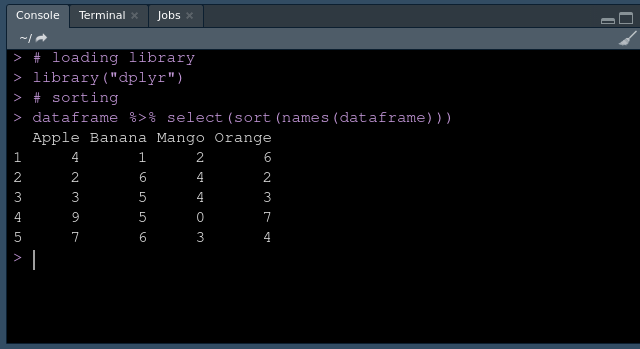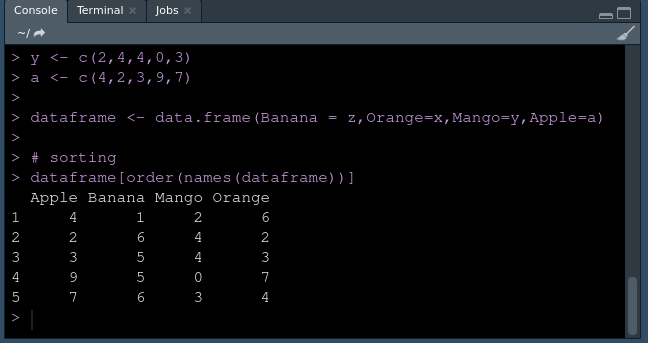Related Articles

# Sort DataFrame by column name in R

• Last Updated : 26 Mar, 2021

Sorting is the process of ordering items. It can be ascending order, descending order, alphabetical order, numerical order. To sort a DataFrame by column name in R programming, we can use various methods as discussed below. To get a better understanding of how to sort DataFrame by column name, let’s take some examples.

Example:

Let’s suppose we have the following dataset with column names as English alphabets and tuples are integer values. Now we want to sort the column by column name in alphabetical order.

After sorting DataFrame by column name it should look alike this:

### Method 1: Using dplyr

dplyr is used to manipulate the DataFrame and names is used to set or get t the object name in R. To use dplyr, it needs to be installed explicitly.

Approach

• Import library
• Create data frame
• Sort the DataFrame using sort function and pass the DataFrame name as an argument.

Syntax:

DataFrame %>% select(sort(names(DataFrame)))

• Display sorted dataframe

Example:

## R

 `#Sort DataFrame by column name in R`` ` `# Creating a dataset.``z <- ``c``(1,6,5,5,6)``x <- ``c``(6,2,3,7,4)``y <- ``c``(2,4,4,0,3)``a <- ``c``(4,2,3,9,7)`` ` `dataframe <- ``data.frame``(Banana = z,Orange=x,Mango=y,Apple=a)`` ` `# install dplyr package``install.packages``(``"dplyr"``)`` ` `# loading library``library``(``"dplyr"``)`` ` `# sorting ``dataframe %>% ``select``(``sort``(``names``(dataframe)))``dataframe`

Output:Sorted DataFrame

### Method 2: Using order

We can use the order function to sort the columns by column name.

Syntax:

order(names(dataframe))

Approach

• Create dataframe
• Pass the names of columns in order function
• Save the sorted data
• Display result

Program:

## R

 `#Sort DataFrame by column name in R`` ` `# Creating a dataset.``z <- ``c``(1,6,5,5,6)``x <- ``c``(6,2,3,7,4)``y <- ``c``(2,4,4,0,3)``a <- ``c``(4,2,3,9,7)`` ` `dataframe <- ``data.frame``(Banana = z,Orange=x,Mango=y,Apple=a)`` ` `# sorting ``dataframe[``order``(``names``(dataframe))]`

Output:Sorted dataframe

My Personal Notes arrow_drop_up